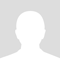# Understanding How Fractions Work

It is very important, in the beginning of fraction work, to explain carefully to students how a fraction is set up -- what the numerator is indicating, what the denominator is indicating, and what the line between them indicates. I believe that this point is the crux of many problems that students encounter in their study of elementary school (and even high school) math. They have never understood that a fraction means that the numerator is being divided by the denominator, and that produces a decimal that is equal to the fraction. They do not realize that a fraction such as 1/2 and a decimal such as 0.5 are equal amounts, just expressed in different forms.

From there, confusion only multiplies. It is important to teach that the line in a fraction means (primarily) "divided by." It can also mean "per," or "for every," or "out of," but primarily, the line in a fraction means "divided by." Thus, students need to know how to divide. But there again, a problem. Many students have not memorized their division facts in such as way as to be able to divide, say, 72 by 8. So at this point, they are stymied.

I hope you can see that it is of great importance to have students memorize their basic addition, subtraction, multiplication, and division facts by, say the end of 4th grade for sure. That way, if they have these memorized, they will be able to "breeze through" any more advanced problems -- because surely those advanced problems are going to require the student to use their skills in addition, subtraction, multiplication, and division -- the four basic processes or arithmetic.\$20p/h

Judy L.

Education Specialist, Patient and Knowledgeable

200+ hours